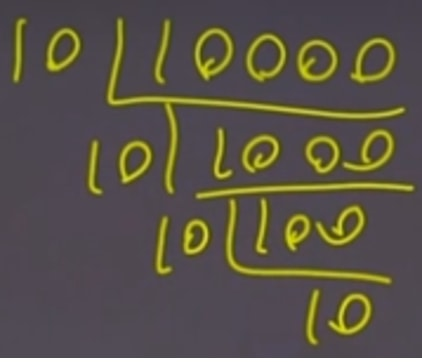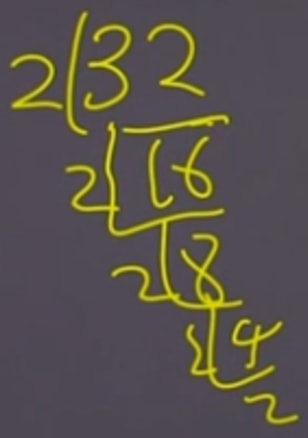# Evaluating and simplifying radicals

## How to evaluate radicals

When we work with the radical sign, what are we dealing with? We'll learn the steps to simplifying radicals so that we can get the final answer to math problems.

## How to simplify radicals

There are four steps you should keep in mind when you try to evaluate radicals. It's a little similar to how you would estimate square roots without a calculator.

1. Find the number under the radical sign's prime factorization. You'll usually start with 2, which is the first prime number, and then you can move on to using numbers such as 3 and 5. Do this until the original number is now completely made up of prime numbers.

2. Figure out what the index of the radical is. If you just see a square root symbol, keep in mind that this is actually an index of 2. For other indices, there should be a tiny little number right before the radical sign that will indicate what the index is. This is the number that will tell you how many repeated prime numbers you'll need to have after factorization in order to move that number inside the radical to outside the radical. If you've got a cube root for example, you'll need a prime number to be found three times through prime factorization in order to move it outside.

3. Now move the groups of numbers from inside the radical sign to outside. If you're working with a cube root and under the radical sign you're left with 3, 3, 3, 2, 11 after prime factorization, you can move the 3's out from the sign. However, you'll only write it once as a group. If you've got prime numbers that can't be move outside, leave them under the radical sign.

4. Clean up what you've got through simplifying. If you've moved more than one group of numbers out, multiply what you've moved outside together. Do the same for the prime numbers you've got left inside the radical. Simplifying expressions is the last step when you evaluate radicals. Then you'll get your final answer!

Don't worry that this isn't super clear after reading through the steps. You'll get a clearer idea of this after following along with the example questions below.

## Example problems

Question:

Evaluate the radicals

${^4}\sqrt{10000}$

Solution:

Firstly, let's do some factorization. Perform a long division to see what number you can pull out. It's most obvious that you can pull out 10's due to the original number being 10,000.Factorization with long division

${^4}\sqrt{10\bullet10\bullet10\bullet10}$

We know that this is a $4^{th}$ root since there's a little 4 right outside the radical sign to indicate this index. We have four 10's under the radical sign. We can take out the 10 and get the final answer:

10

Question:

Evaluate the radicals

${^5}\sqrt{\frac{1}{32}}$

Solution:

Firstly, the following is true:

${^5}\sqrt{1} = 1$

Then we are now free to deal with the denominator in this question. Perform long division on 32 to factorize it.Perform long division for factorization

${^5}\sqrt{2\bullet2\bullet2\bullet2\bullet2}$

Since it's a $5^{th}$ root, and we have five 2's under the radical sign, we can take out the 2 and get a final answer of:

$\frac{1}{2}$

## Using calculator to evaluate radicals

An online calculator for simplifying radicals you can use can be found here. In your tests and exams, you will have to evaluate radicals without the use of a calculator, so make sure you've firmly understood the four steps in this lesson.

Looking to move on? Learn how to do operations with radicals, add and subtract them, and learn about converting entire radicals to mixed radicals.

### Evaluating and simplifying radicals

To simplify radicals, we will need to find the prime factorization of the number inside the radical sign first. Then, move each group of prime factors outside the radical according to the index. The last step is to simplify the expression by multiplying the numbers both inside and outside the radical sign.

#### Lessons

• Introduction
a)
How to simplify radicals?

• 1.
Evaluate the radicals
a)
${^4}\sqrt{10000}$

b)
${^5}\sqrt{1/32}$

• 2.
Using calculator to evaluate
a)
${^7}\sqrt{4365}$

b)
-${^5}\sqrt{234}$